【一猫汽车网】2016年9月9日，你从北方来，带着炫酷的外观、明媚的阳光、湛蓝的天空和那让人心旷神怡养眼的绿，令人欣羡不已！我同样渴望北方的初秋，所以你携车队千里迢迢从北京赶来上海，告诉我已经发生和将要发生的事，而你也将在此地逗留，因为江南正值烟雨中…

【一猫汽车网】2016年9月9日，你从北方来，带着炫酷的外观、明媚的阳光、湛蓝的天空和那让人心旷神怡养眼的绿，令人欣羡不已！我同样渴望北方的初秋，所以你携车队千里迢迢从北京赶来上海，告诉我已经发生和将要发生的事，而你也将在此地逗留，因为江南正值烟雨中…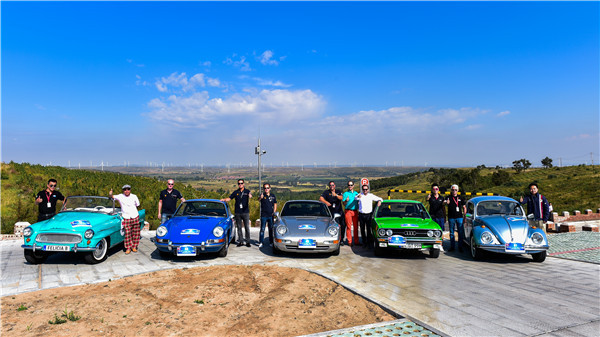稍前两日，身在魔都，温度适宜，信步披薄纱，微眠盖薄被，三两场雨过后，以为秋至。当日却晴空万里、酷热如暑，颇有一番忽闻你来我热情相迎的意味。且魔都的人民也非常热情，瞬间被众老爷车的外观深深地吸引，争相拍照留念，害怕稍稍迟疑便错过了东方明珠前这复古的情景。当时我多想着一身旗袍、撑一把油纸伞与你依偎，你说你是那个时代的产物，而我可能会告诉你我曾见过你在电影里，只是你与魔都相遇太短，半日便要分离。幸好，接下来的三天我都将与你相伴，因为我想与你一起领略江南秋日的美，你看那微黄的树叶、盛开的紫薇都是为你的到来而准备。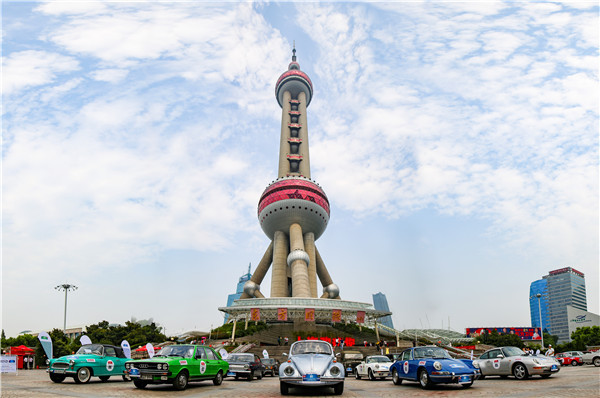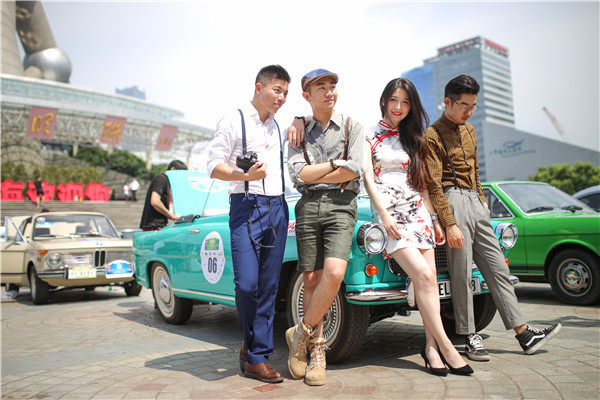车在路上飞驰，不到三个小时我们便到了乌镇——一个你想在此创造回忆而我却是来寻找回忆的地方。记得那年我们都还年轻，有很灿烂的笑容和用不完的精力，一时兴起一行五人便在春日的一个小长假简单收拾了行李转了三次车来到了乌镇，我们一路高歌、嬉笑、停下拍照，仿佛我们才是乌镇的春天里最快乐的小鸟。而你来此，包下整个西栅尽情狂欢，估计是想为随行的人创造更多、更美好的回忆吧！你用心计划，带着我们去吃长街宴、在酒吧里为我们腾出空间、小桥上为我们点几盏灯，举杯换盏时、觥筹交错间、灯光交汇处无一不透露着你是个有故事的人，而乌镇便是在听你讲故事。此时，你有故事他有酒，真是一大幸事。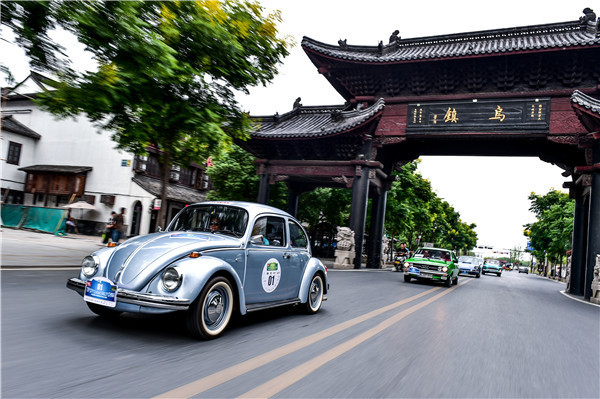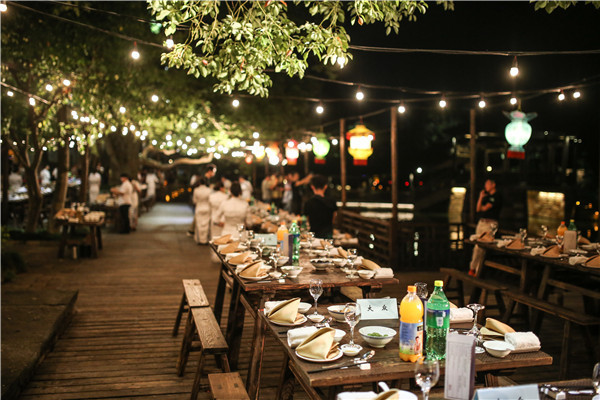9月10日，故事还没说完，你却要继续赶路了…

下一站将与谁遇见？我想你应该是满心欢喜的，因为确是老友相见，格外期待！这一路也随着你的心情激动着、跳跃着，那山上零星散落着的红色彼岸花一闪而过却仿佛开了漫山遍野，就像你的几根兴奋的神经，可以瞬间把欢乐传递给所有的参与者。大巴车上少数没有跟车的媒体老师们一路开着玩笑“你看！行李在飞！”“哎呀！刚刚的一颠，我的头重重地撞上了车顶”，然后哄堂大笑，任凭汽车在山路上颠簸，在急速地过了好多个弯道后，我知道千岛湖已然近在咫尺了。你一袭白衣，长袖善舞，我满身青黛，水墨江南，我们仿佛融入了这幅水墨画中，被无端陶醉。此时也适时地下起了小雨，曾想有多少人在秋天赶来，只为凭栏一睹江南的烟雨呢？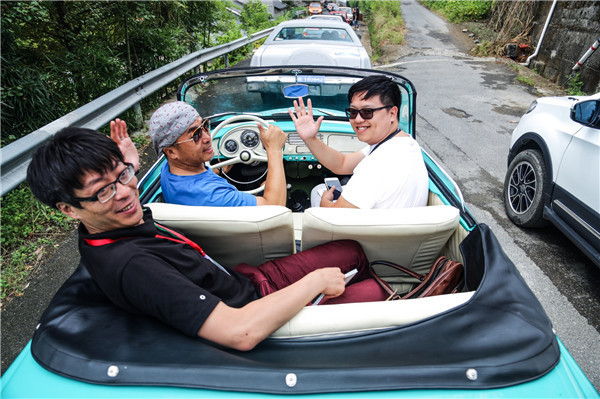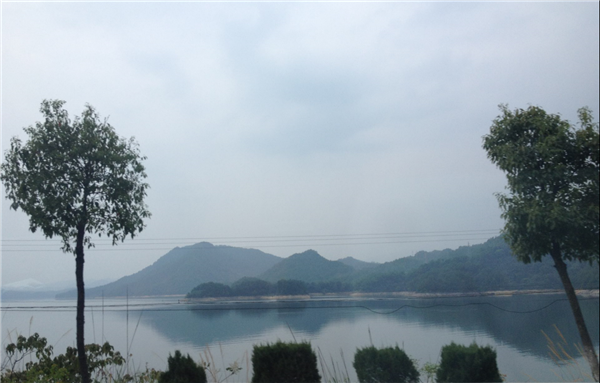9月11日，每个故事都在等一个结局…

今天是你江南之行最后的日子，许是不舍你的离去，所以早晨开始雨下的便越发地大了。而今天也是与你亲密接触的日子，因为今天我跟车，辛苦是可以预见的，但心里是喜悦的。有人说：“如果你喜欢上一个人，便会去走他走过的路、看他看过的风景、吃他大呼好吃的东西”，因为你想了解关于他的一切，而我…只是想与你携手看遍这剩下的风景。

你豁达却不失严谨、开朗却不大意、追求精准却不拘泥于小节、富有爱心却有很强的时间观念，或许正是因为此，所以你在威坪中学吸引了大批来围观的同学，你让他们画你、读你、与你合照、和你交谈，互通彼此；在隧道口对被沿途美景吸引而随意变道、减速的驾驶员加以严厉的批评；在追求速度和精准的时候不忘问些幽默的问题来活跃气氛；路过开满格桑花的山坡时为了满足女生对美的追求停下车让我尽情地拍照，即使你已被淋湿。突然间明白了你此行的目的，你是想让大家感受驾乘的乐趣的同时了解汽车对于时代的意义。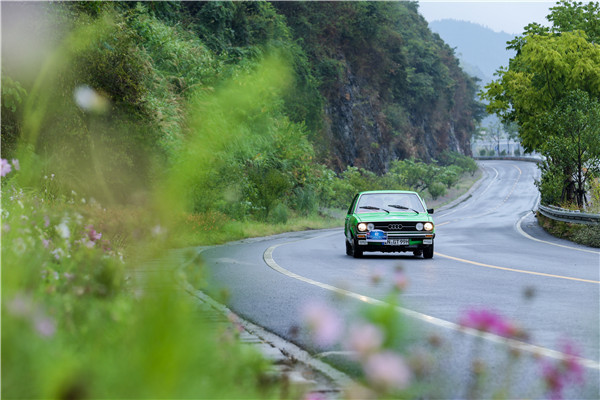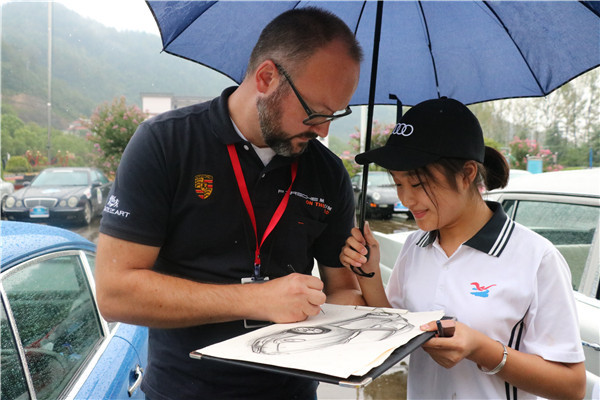原来你就是我们受大众汽车集团（中国）之邀参加的第三届“中国国际名城经典汽车拉力赛（China Rally）”，令人欣喜的是大众汽车集团（中国）参赛的车队也在本次活动中取得了骄人的成绩：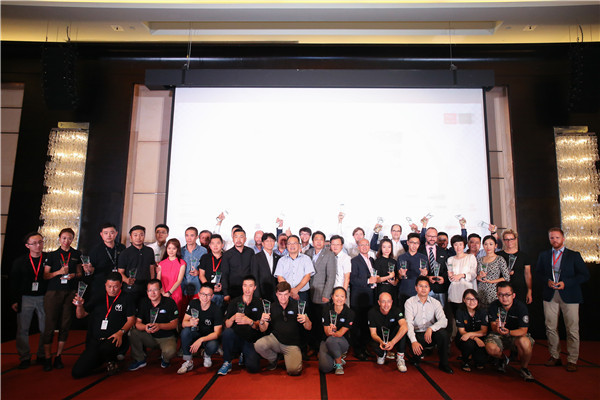你赞叹江南的烟雨、喜欢江南的美，所以你还会来的是吧？你说：“明年9月下旬见！”

|分享

•已阅！握爪
•我手滑为你点赞
•128个赞！
•不明觉厉
•阅后既醉
•有钱！任性
•照片太美，我不敢看
•干货！杠杠的
•高大上
•膜拜中！
•请接受我的膝盖
•猫哥/猫妹么么哒
•神吐槽
•我只笑笑不说话
•我想静静
•窒息
##### 热度排行• 阿斯顿·马丁
• 奥迪
• 阿尔法罗密欧
• ALPINA
• 爱驰
• AC Schnitzer
• AITO
• ARCFOX极狐
• AUXUN傲旋

• 巴博斯
• 宝骏
• 宝马
• 保时捷
• 北汽制造
• 奔驰
• 奔腾
• 本田
• 比亚迪
• 标致
• 别克
• 宾利
• 布加迪
• 北汽威旺
• 北京
• 北汽绅宝
• 北汽幻速
• 北汽新能源
• 宝沃
• 比速汽车
• 北汽道达
• 比德文汽车
• 铂驰
• 北汽昌河
• 百智新能源
• 宝骐汽车
• 比克汽车
• 博速

• 昌河
• 长安
• 长城
• 长安商用
• 成功汽车
• 长江EV
• 长安轻车型
• 长安欧尚
• 长安凯程
• 车驰汽车
• 橙仕
• 创维汽车

• 大众
• 道奇
• 东风
• 东风风神
• 东风小康
• 东南
• DS
• 东风风行
• 东风风度
• 东风风光
• 电咖
• 大乘汽车
• 大运
• 东风富康
• 东风·瑞泰特
• 东风新能源
• 东风御风

• 法拉利
• 菲亚特
• 丰田
• 福迪
• 福特
• 福田汽车
• 福汽启腾
• 风诺
• 飞碟汽车
• 飞凡汽车

• GMC
• 广汽传祺
• 广汽吉奥
• 观致
• 国金汽车
• 国机智骏
• 高合汽车
• 广汽埃安

• 哈飞
• 海格
• 海马
• 华泰
• 黄海
• 恒天
• 红旗
• 哈弗
• 华颂
• 华凯
• 华泰新能源
• 汉腾汽车
• 华利
• 红星汽车
• HYCAN合创
• 汉龙汽车
• 恒润汽车
• 宏远汽车
• 华晨新日
• 华骐
• 华梓汽车

• Icona
• IMSA英飒

• Jeep
• 江淮
• 江铃
• 捷豹
• 金杯
• 九龙
• 吉利汽车
• 金旅
• 金龙
• 江铃集团轻汽
• 江铃集团新能源
• 君马汽车
• 捷途
• 钧天
• 捷达
• 几何汽车
• 极氪
• 捷尼赛思
• 金冠汽车

• 开瑞
• 凯迪拉克
• 科尼赛克
• 克莱斯勒
• KTM
• 卡威
• 凯翼
• 康迪
• 康迪电动汽车集团
• 开沃汽车
• 卡升
• 克蒂汽车
• 克慕勒

• 猎豹汽车
• 兰博基尼
• 劳斯莱斯
• 雷克萨斯
• 雷诺
• 理念
• 力帆
• 莲花汽车
• 林肯
• 铃木
• 陆风
• 路虎
• 路特斯
• 领志
• 领克
• 零跑汽车
• Lorinser
• LOCAL MOTORS
• 领途汽车
• 理想汽车
• 罗夫哈特
• 力帆汽车
• LEVC
• LIMGENE凌际
• LUMMA
• 莱茵汽车
• 岚图汽车
• 凌宝汽车
• 龙程汽车

• MG
• MINI
• 马自达
• 玛莎拉蒂
• 迈凯伦
• 摩根
• 迈莎锐
• 迈迈
• 摩登汽车

• 纳智捷
• 哪吒汽车

• 讴歌
• 欧宝
• 欧朗
• 欧拉
• 欧尚汽车

• 帕加尼
• Polestar极星
• 朋克汽车

• 奇瑞
• 启辰
• 起亚
• 前途
• 庆铃汽车
• 骐铃汽车
• 乔治·巴顿

• 日产
• 荣威
• 瑞麒汽车
• 如虎
• 瑞驰
• 瑞驰新能源
• 容大智造
• RAM
• 睿蓝汽车

### S

• 上汽大通
• smart
• 三菱
• 双环
• 双龙
• 斯巴鲁
• 斯柯达
• 萨博
• 思铭
• 赛麟
• SWM斯威汽车
• SRM鑫源
• 思皓
• SHELBY
• 上喆
• SERES赛力斯
• SONGSAN MOTORS
• 速达
• 申龙客车
• 神州

• TESLA
• 泰卡特
• 腾势
• 特斯拉
• 天际汽车
• TECHART
• 坦克

• 威麟
• 威兹曼
• 沃尔沃
• 五菱汽车
• 五十铃
• 潍柴英致
• WEY
• 蔚来
• 威马汽车
• WALD
• 瓦滋
• 伟昊汽车
• 魏牌

• 现代
• 雪佛兰
• 雪铁龙
• 西雅特
• 新特汽车
• 小鹏汽车
• 新宝骏
• 小虎
• 小猬汽车
• 晓奥汽车

• 野马汽车
• 一汽
• 依维柯
• 英菲尼迪
• 永源
• 驭胜
• 云度
• 宇通客车
• 云雀汽车
• 远程汽车
• 一汽凌河
• 雅升汽车
• 野马新能源
• 银隆新能源
• 御捷
• 裕路

• 中华
• 中兴
• 众泰
• 知豆
• 之诺
• 正道汽车
• 智点汽车
• 智己汽车
• 中国重汽VGV
• A
• B
• C
• D
• E
• F
• G
• H
• I
• J
• K
• L
• M
• N
• O
• P
• Q
• R
• S
• T
• U
• V
• W
• X
• Y
• Z

• 阿斯顿·马丁
• 奥迪
• 阿尔法罗密欧
• ALPINA
• 爱驰
• AC Schnitzer
• AITO
• ARCFOX极狐
• AUXUN傲旋

• 巴博斯
• 宝骏
• 宝马
• 保时捷
• 北汽制造
• 奔驰
• 奔腾
• 本田
• 比亚迪
• 标致
• 别克
• 宾利
• 布加迪
• 北汽威旺
• 北京
• 北汽绅宝
• 北汽幻速
• 北汽新能源
• 宝沃
• 比速汽车
• 北汽道达
• 比德文汽车
• 铂驰
• 北汽昌河
• 百智新能源
• 宝骐汽车
• 比克汽车
• 博速

• 昌河
• 长安
• 长城
• 长安商用
• 成功汽车
• 长江EV
• 长安轻车型
• 长安欧尚
• 长安凯程
• 车驰汽车
• 橙仕
• 创维汽车

• 大众
• 道奇
• 东风
• 东风风神
• 东风小康
• 东南
• DS
• 东风风行
• 东风风度
• 东风风光
• 电咖
• 大乘汽车
• 大运
• 东风富康
• 东风·瑞泰特
• 东风新能源
• 东风御风

• 法拉利
• 菲亚特
• 丰田
• 福迪
• 福特
• 福田汽车
• 福汽启腾
• 风诺
• 飞碟汽车
• 飞凡汽车

• GMC
• 广汽传祺
• 广汽吉奥
• 观致
• 国金汽车
• 国机智骏
• 高合汽车
• 广汽埃安

• 哈飞
• 海格
• 海马
• 华泰
• 黄海
• 恒天
• 红旗
• 哈弗
• 华颂
• 华凯
• 华泰新能源
• 汉腾汽车
• 华利
• 红星汽车
• HYCAN合创
• 汉龙汽车
• 恒润汽车
• 宏远汽车
• 华晨新日
• 华骐
• 华梓汽车

• Icona
• IMSA英飒

• Jeep
• 江淮
• 江铃
• 捷豹
• 金杯
• 九龙
• 吉利汽车
• 金旅
• 金龙
• 江铃集团轻汽
• 江铃集团新能源
• 君马汽车
• 捷途
• 钧天
• 捷达
• 几何汽车
• 极氪
• 捷尼赛思
• 金冠汽车

• 开瑞
• 凯迪拉克
• 科尼赛克
• 克莱斯勒
• KTM
• 卡威
• 凯翼
• 康迪
• 康迪电动汽车集团
• 开沃汽车
• 卡升
• 克蒂汽车
• 克慕勒

• 猎豹汽车
• 兰博基尼
• 劳斯莱斯
• 雷克萨斯
• 雷诺
• 理念
• 力帆
• 莲花汽车
• 林肯
• 铃木
• 陆风
• 路虎
• 路特斯
• 领志
• 领克
• 零跑汽车
• Lorinser
• LOCAL MOTORS
• 领途汽车
• 理想汽车
• 罗夫哈特
• 力帆汽车
• LEVC
• LIMGENE凌际
• LUMMA
• 莱茵汽车
• 岚图汽车
• 凌宝汽车
• 龙程汽车

• MG
• MINI
• 马自达
• 玛莎拉蒂
• 迈凯伦
• 摩根
• 迈莎锐
• 迈迈
• 摩登汽车

• 纳智捷
• 哪吒汽车

• 讴歌
• 欧宝
• 欧朗
• 欧拉
• 欧尚汽车

• 帕加尼
• Polestar极星
• 朋克汽车

• 奇瑞
• 启辰
• 起亚
• 前途
• 庆铃汽车
• 骐铃汽车
• 乔治·巴顿

• 日产
• 荣威
• 瑞麒汽车
• 如虎
• 瑞驰
• 瑞驰新能源
• 容大智造
• RAM
• 睿蓝汽车

### S

• 上汽大通
• smart
• 三菱
• 双环
• 双龙
• 斯巴鲁
• 斯柯达
• 萨博
• 思铭
• 赛麟
• SWM斯威汽车
• SRM鑫源
• 思皓
• SHELBY
• 上喆
• SERES赛力斯
• SONGSAN MOTORS
• 速达
• 申龙客车
• 神州

• TESLA
• 泰卡特
• 腾势
• 特斯拉
• 天际汽车
• TECHART
• 坦克

• 威麟
• 威兹曼
• 沃尔沃
• 五菱汽车
• 五十铃
• 潍柴英致
• WEY
• 蔚来
• 威马汽车
• WALD
• 瓦滋
• 伟昊汽车
• 魏牌

• 现代
• 雪佛兰
• 雪铁龙
• 西雅特
• 新特汽车
• 小鹏汽车
• 新宝骏
• 小虎
• 小猬汽车
• 晓奥汽车

• 野马汽车
• 一汽
• 依维柯
• 英菲尼迪
• 永源
• 驭胜
• 云度
• 宇通客车
• 云雀汽车
• 远程汽车
• 一汽凌河
• 雅升汽车
• 野马新能源
• 银隆新能源
• 御捷
• 裕路

• 中华
• 中兴
• 众泰
• 知豆
• 之诺
• 正道汽车
• 智点汽车
• 智己汽车
• 中国重汽VGV
• A
• B
• C
• D
• E
• F
• G
• H
• I
• J
• K
• L
• M
• N
• O
• P
• Q
• R
• S
• T
• U
• V
• W
• X
• Y
• Z

• 阿斯顿·马丁
• 奥迪
• 阿尔法罗密欧
• ALPINA
• 爱驰
• AC Schnitzer
• AITO
• ARCFOX极狐
• AUXUN傲旋

• 巴博斯
• 宝骏
• 宝马
• 保时捷
• 北汽制造
• 奔驰
• 奔腾
• 本田
• 比亚迪
• 标致
• 别克
• 宾利
• 布加迪
• 北汽威旺
• 北京
• 北汽绅宝
• 北汽幻速
• 北汽新能源
• 宝沃
• 比速汽车
• 北汽道达
• 比德文汽车
• 铂驰
• 北汽昌河
• 百智新能源
• 宝骐汽车
• 比克汽车
• 博速

• 昌河
• 长安
• 长城
• 长安商用
• 成功汽车
• 长江EV
• 长安轻车型
• 长安欧尚
• 长安凯程
• 车驰汽车
• 橙仕
• 创维汽车

• 大众
• 道奇
• 东风
• 东风风神
• 东风小康
• 东南
• DS
• 东风风行
• 东风风度
• 东风风光
• 电咖
• 大乘汽车
• 大运
• 东风富康
• 东风·瑞泰特
• 东风新能源
• 东风御风

• 法拉利
• 菲亚特
• 丰田
• 福迪
• 福特
• 福田汽车
• 福汽启腾
• 风诺
• 飞碟汽车
• 飞凡汽车

• GMC
• 广汽传祺
• 广汽吉奥
• 观致
• 国金汽车
• 国机智骏
• 高合汽车
• 广汽埃安

• 哈飞
• 海格
• 海马
• 华泰
• 黄海
• 恒天
• 红旗
• 哈弗
• 华颂
• 华凯
• 华泰新能源
• 汉腾汽车
• 华利
• 红星汽车
• HYCAN合创
• 汉龙汽车
• 恒润汽车
• 宏远汽车
• 华晨新日
• 华骐
• 华梓汽车

• Icona
• IMSA英飒

• Jeep
• 江淮
• 江铃
• 捷豹
• 金杯
• 九龙
• 吉利汽车
• 金旅
• 金龙
• 江铃集团轻汽
• 江铃集团新能源
• 君马汽车
• 捷途
• 钧天
• 捷达
• 几何汽车
• 极氪
• 捷尼赛思
• 金冠汽车

• 开瑞
• 凯迪拉克
• 科尼赛克
• 克莱斯勒
• KTM
• 卡威
• 凯翼
• 康迪
• 康迪电动汽车集团
• 开沃汽车
• 卡升
• 克蒂汽车
• 克慕勒

• 猎豹汽车
• 兰博基尼
• 劳斯莱斯
• 雷克萨斯
• 雷诺
• 理念
• 力帆
• 莲花汽车
• 林肯
• 铃木
• 陆风
• 路虎
• 路特斯
• 领志
• 领克
• 零跑汽车
• Lorinser
• LOCAL MOTORS
• 领途汽车
• 理想汽车
• 罗夫哈特
• 力帆汽车
• LEVC
• LIMGENE凌际
• LUMMA
• 莱茵汽车
• 岚图汽车
• 凌宝汽车
• 龙程汽车

• MG
• MINI
• 马自达
• 玛莎拉蒂
• 迈凯伦
• 摩根
• 迈莎锐
• 迈迈
• 摩登汽车

• 纳智捷
• 哪吒汽车

• 讴歌
• 欧宝
• 欧朗
• 欧拉
• 欧尚汽车

• 帕加尼
• Polestar极星
• 朋克汽车

• 奇瑞
• 启辰
• 起亚
• 前途
• 庆铃汽车
• 骐铃汽车
• 乔治·巴顿

• 日产
• 荣威
• 瑞麒汽车
• 如虎
• 瑞驰
• 瑞驰新能源
• 容大智造
• RAM
• 睿蓝汽车

### S

• 上汽大通
• smart
• 三菱
• 双环
• 双龙
• 斯巴鲁
• 斯柯达
• 萨博
• 思铭
• 赛麟
• SWM斯威汽车
• SRM鑫源
• 思皓
• SHELBY
• 上喆
• SERES赛力斯
• SONGSAN MOTORS
• 速达
• 申龙客车
• 神州

• TESLA
• 泰卡特
• 腾势
• 特斯拉
• 天际汽车
• TECHART
• 坦克

• 威麟
• 威兹曼
• 沃尔沃
• 五菱汽车
• 五十铃
• 潍柴英致
• WEY
• 蔚来
• 威马汽车
• WALD
• 瓦滋
• 伟昊汽车
• 魏牌

• 现代
• 雪佛兰
• 雪铁龙
• 西雅特
• 新特汽车
• 小鹏汽车
• 新宝骏
• 小虎
• 小猬汽车
• 晓奥汽车

• 野马汽车
• 一汽
• 依维柯
• 英菲尼迪
• 永源
• 驭胜
• 云度
• 宇通客车
• 云雀汽车
• 远程汽车
• 一汽凌河
• 雅升汽车
• 野马新能源
• 银隆新能源
• 御捷
• 裕路

• 中华
• 中兴
• 众泰
• 知豆
• 之诺
• 正道汽车
• 智点汽车
• 智己汽车
• 中国重汽VGV
• A
• B
• C
• D
• E
• F
• G
• H
• I
• J
• K
• L
• M
• N
• O
• P
• Q
• R
• S
• T
• U
• V
• W
• X
• Y
• Z